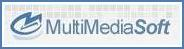﻿ Tutorials > How to define an equalizerCopyright © 2008-2019 MultiMedia Soft How to define an equalizerActive Sound Editor comes with an embedded equalizer, implemented through a set of methods of the Effects COM object, which allows setting up a pool of equalizer bands; each equalizer band works on a different frequency and the current available range of frequencies is from 80 to 16000 (expressed in Hertz).

Each equalizer band is always identified by its frequency expressed in Hertz: it's not possible defining more than one band having the same frequency. Note that a band's frequency cannot exceed one-third of the frequency of the sound under editing so, if you have loaded a sound with a frequency of 44100 Hz, the maximum allowed band's frequency will be 14700 Hz (14700 * 3 = 44100); in order to reach a band's frequency of 16000 Hz, you must load sounds whose frequency is at least 48000 Hz.

The first step for creating an equalizer band and for adding it to the control's equalizer is to call the Effects.EqualizerBandAdd method. Each band can be removed at a later time using the Effects.EqualizerBandRemove method and its settings can be retrieved through the Effects.EqualizerBandGetParams method and modified through the Effects.EqualizerBandSetGain and Effects.EqualizerBandSetParams methods.

Existing bands can be enumerated using the Effects.EqualizerBandGetCount and Effects.EqualizerBandGetFrequency methods.

Once the equalizer has been defined, there is the possibility to store its bands and their respective settings into a destination file through the Effects.EqualizerSave method and retrieved at a later time through the Effects.EqualizerLoad method. Equalizer settings are stored into a XML based file (usually with .EDJ extension) and has a structure like the sample below:

<?xml version="1.0" ?>

<Equalizer>

<Bands>

<Band FreqInHz="80" BandWidth="12" GainIndB="-5.200000">0</Band>

<Band FreqInHz="170" BandWidth="12" GainIndB="3.750000">1</Band>

<Band FreqInHz="310" BandWidth="12" GainIndB="0.000000">2</Band>

<Band FreqInHz="600" BandWidth="12" GainIndB="-4.500000">3</Band>

<Band FreqInHz="1000" BandWidth="12" GainIndB="-3.000000">4</Band>

<Band FreqInHz="3000" BandWidth="12" GainIndB="1.125000">5</Band>

<Band FreqInHz="6000" BandWidth="12" GainIndB="5.250000">6</Band>

<Band FreqInHz="12000" BandWidth="12" GainIndB="6.750000">7</Band>

<Band FreqInHz="14000" BandWidth="12" GainIndB="-3.120000">8</Band>

</Bands>

</Equalizer>

As you can see each band has a progressive number (in the case above from 0 to 8) and is identified by the frequency stored inside the FreqInHz attribute (expressed in Hertz) while its parameters are defined inside the BandWidth (expressed in semi-tones) and GainIndB (expressed in dB) attributes.

EDJ format is also used by our Active DJ Studio component.

A further feature for setting up the equalizer is to load bands containing presets as defined by WinAmp™ using the Effects.EqualizerLoadPresets method.

Gain of available bands can be reset to 0 dB using the Effects.EqualizerReset method.

Once the equalizer has been setup, you can apply its setting to the sound under editing using the Effects.EqualizerApply method.

The creation and use of equalizer bands requires DirectX version 8 or higher to be installed on the target system: you can verify the availability of this DirectX version calling the GetDirectSoundVersion method.